# Imbedding theorems for Orlicz-Sobolev spaces

(diff) ← Older revision | Latest revision (diff) | Newer revision → (diff)
Establishing imbedding theorems goes back to T.K. Donaldson and N.S. Trudinger, and R.A. Adams (see [a4], [a10], [a2]). For the sake of simplicity, consider the spacewith an-function(cf. Orlicz–Sobolev space), where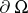is sufficiently smooth (a Lipschitz boundary, for instance; see [a1]). Define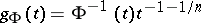,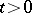. The case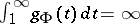corresponds to the sublimiting case for usual Sobolev spaces (cf. also Sobolev space) and in this case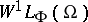is imbedded into, where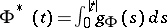,. The case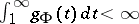corresponds to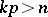for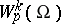. If one defines the generalized Hölder spaces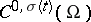, whereis a increasing continuous function on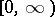such that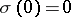, as the space of continuous functions on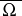, for which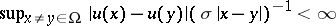, then the target space for the imbeddings is a Hölder space of this type with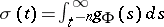.
A (partial) ordering of such functions(and of-functions) can be introduced in the following way: Ifand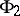are-functions, then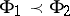if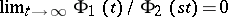for every. Further,if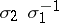is a function of the same type. If nowis imbedded into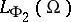and, thenis compactly imbedded into, and ifis imbedded into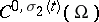and, thenis compactly imbedded into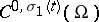.
The sublimiting case was handled also by the method of Fourier analysis (cf. e.g. [a9], [a8]), by considering potential Orlicz–Sobolev spaces (nevertheless, in this case non-reflexive spaces are excluded). The problem of the best target space in the scale of Orlicz spaces has been dealt with in, e.g., [a3]). Recently (1998), logarithmic Sobolev spaces, which are nothing but Orlicz–Sobolev spaces with generating function of the type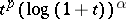, tuning the scale of Sobolev spaces, have been used in connection with limiting imbeddings into exponential Orlicz spaces and/or logarithmic Lipschitz spaces (see, e.g., [a7], [a5], [a6]).